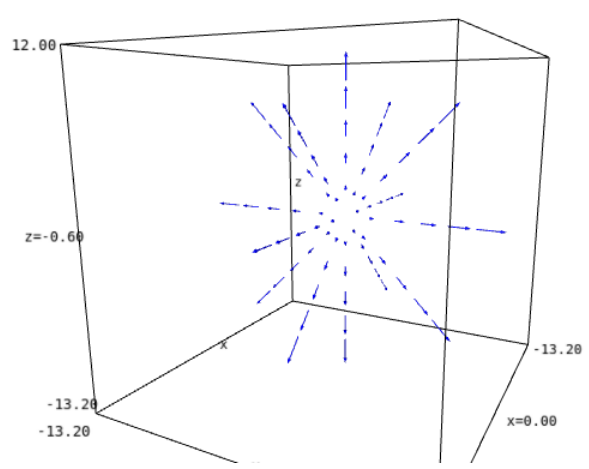# Revision history [back]

Instead of plot_vector_field3d, use the method plot of vector fields:

vf.plot(chart=c_cart, chart_domain=c_spher, ranges={r: (2, 10)}, scale=0.2, width=2)


The output isSee the documentation of VectorField.plot() for explanations and more examples.

Instead of plot_vector_field3d, use the method plot of vector fields:

vf.plot(chart=c_cart, chart_domain=c_spher, ranges={r: (2, 10)}, scale=0.2, width=2)


The output isSee the documentation of VectorField.plot() for explanations and more examples.

Side note: there are some typos in your example (E.vectorfield --> E.vector_field, cart --> chart); it should read

vf = E.vector_field((r,0,0), frame=f_spher, chart=c_spher, name="vf")


Besides, instead of f_spher = E.frames(), you can use f_spher = E.spherical_frame().